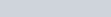ChemTalk

# What is a Buffer Solution?## Core Concepts

This chemistry tutorial introduces you to buffers by learning the definition of a buffer, and the components of acidic and basic buffer solutions and how they react with added acids and bases. In addition, you will learn examples of different potential buffers. Finally, we will touch upon the Henderson Hasselbalch equation. Let’s find out, what is a buffer solution?

## Buffer Solution Definition

In chemistry, the definition of a buffer is a solution that can resist pH change upon the addition of an acid or a base. It consists of a solution of a weak acid and its conjugate base, or vice versa.

A buffer is an extremely useful solution used in acid base chemistry. Sometimes, when solutions mix with a strong acid or base, the pH can change both quickly and dramatically. A buffer solution helps to neutralize some of the added acid or base so that the pH can change more gradually. Buffers achieve this by consisting of components that will react with hydrogen or hydroxide ions so that they cannot affect pH at maximum capacity.

Buffer solutions have limits to how much they can neutralize; once this buffer reaches its capacity, the solution will act as if no buffer is present, and the pH can begin changing dramatically once again.

Analyzing and experimenting with strong acids and bases becomes easier by using buffers. Biocarbonate buffering system, used to regulate the pH of blood, provides an example of real world use of buffers.

## Components of a Buffer Solution

A buffer must contain one of two choices: a weak acid and its conjugate base or a weak base and its conjugate acid. The way that the components are chosen have to do with the desired pH of the solution.

### Acidic Buffer

An acidic buffer has a pH of less than 7; these solutions are made with a weak acid and its conjugate base as a salt. If you want to change the pH of the solution, you can change the ratio between the acid and salt. Additionally, different acids (along with their conjugate salts) can affect the pH in different ways.

Acetic acid and sodium acetate is an example of a weak acid and its conjugate salt. The acid equilibrium is going left; however, if you add sodium acetate, acetate ions are added into solution. Due to Le Chatelier’s Principle, the equilibrium will then switch to the left.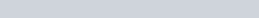### Adding Acid to Acidic Buffer

Keeping the example buffer from above in mind, what would happen if acid was added? The buffer has to work to remove the hydrogen ions from the incoming acid. The acetate ions will combine with these hydrogen ions to create acetic acid. Since acetic acid is a weak acid, the reaction can remove hydrogen ions quicker than the acetic acid can dissociate again; this is why the pH does not change dramatically.

### Adding Base to Acidic Buffer

Again, let’s keep in mind the buffer solution from above. Adding base to interact with this buffer is a bit more complex. The acetic acid will interact with the hydroxide ion from the base which will cause acetate ions to form, along with water; since the hydroxide ions become consumed from this, they will not cause an increase in pH. Additionally, extra hydrogen ions from the dissociation of the acetic acid can also combine with the hydroxide to form water. With these methods, most of the hydroxide ions interact with hydrogen ions to remove the basic presence, but not enough to drastically change the pH.

### Basic (Alkaline) Buffer

A basic buffer has a pH greater than 7; these solutions are made from a weak base and its conjugate acid as a salt. The concentrations of both components should be equal to start; however, like acidic buffers, you can change the pH of the solution by changing the ratio between base and acidic salt.

Ammonia and ammonium chloride is an example of a weak base with its conjugate acid. The equilibrium is going toward the left; however, if you add ammonium chloride, ammonium ions are added into the solution. This time, Le Chatelier’s Principle’s will cause the equilibrium to move even further left.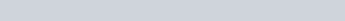### Adding Acid to Basic Buffer

Keeping in mind the example buffer from above, let’s analyze what would happen if acid was added. The hydrogen from the added acid will interact with the ammonia to form ammonium ions. This will cause the removal of many hydrogen ions. Additionally, there are some hydroxide ions in the solution, due to the reaction between ammonia and water; these hydroxide ions will interact with the acidic hydrogens to form more water. These methods will cause the removal of most of the hydrogen ions to remove the acidic presence, which will help buffer the pH change.

### Adding Base to Basic Buffer

What if we add base to the buffer solution from above? The buffer has to work to remove the hydroxide ions from the incoming base. The ammonium ions in the solution will react with the hydroxide ions. Because ammonia is a weak base, the reaction can be reverse when reacting with water, but not all the hydroxide ions will be removed from solution. Due to this, the pH won’t change dramatically.

## Buffer Solution Examples

• Acetic acid & conjugate base: CH3COOH & CH3COO
• Formic acid & conjugate base: HCHO2 & CHO2
• Pyridine & conjugate acid: C5H5N & C5H5H+
• Ammonia & conjugate acid: NH3 & NH4+
• Methylamine & conjugate acid: CH3NH2 & CH3NH3+
• Many biological systems require buffers for balance

## Henderson Hasselbalch Equation

When you create a buffer using a weak acid and its conjugate base, it maintains a buffer pH, and can resist changes to that pH with small additions of acid or base. The Henderson Hasselbalch equation, shown below, uses the pKa of the weak acid to calculate what the buffer pH is. We’ll go into detailed examples using this equation in a separate article.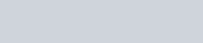## Buffer Solution Practice Problems

Problem 1

You mix together a buffer with final concentrations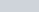acetic acid and. Using the Henderson-Hasselbalch equation, calculate the pH of the buffer. (Acetic acid has a pKa of 4.76).

Problem 2

You have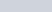of a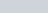solution of benzoic acid, with a pKa of 4.20. How many grams of potassium benzoate (C7H5O2K) do you need to add to make a buffer of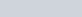?

## Buffer Solution Practice Problem Solutions

1: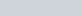2: